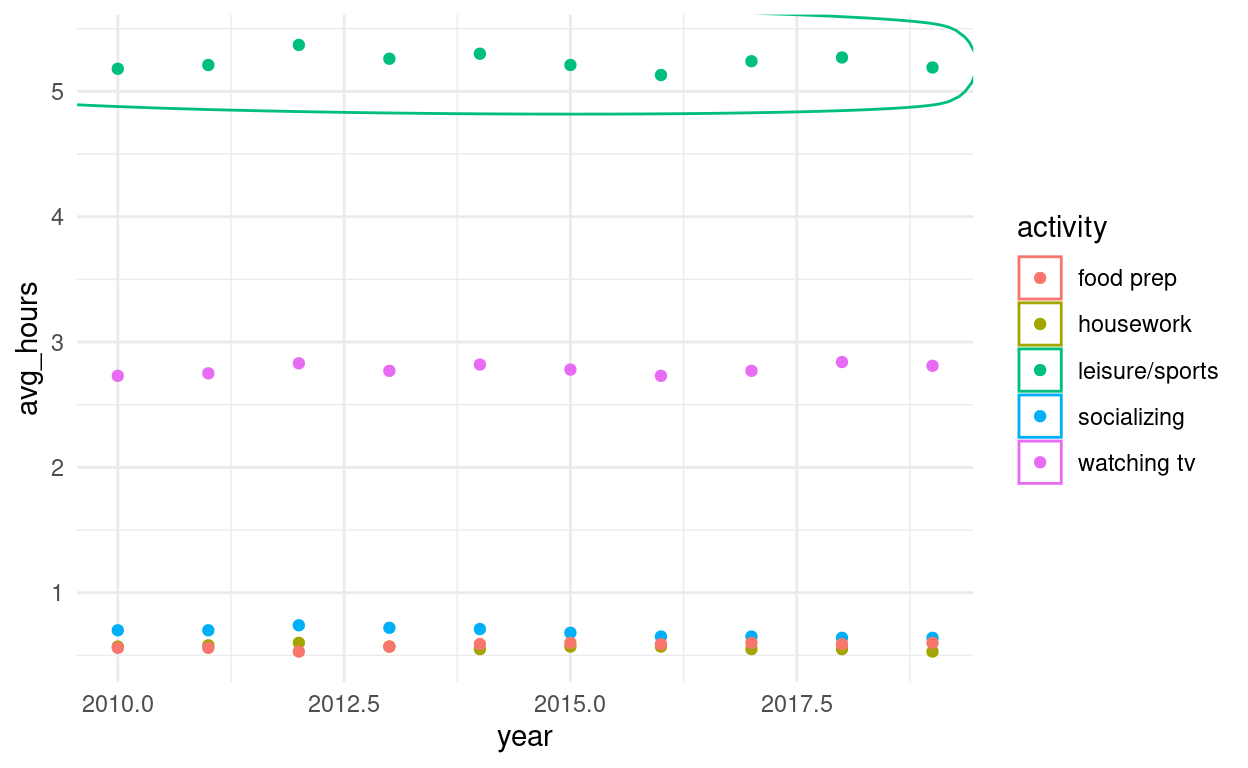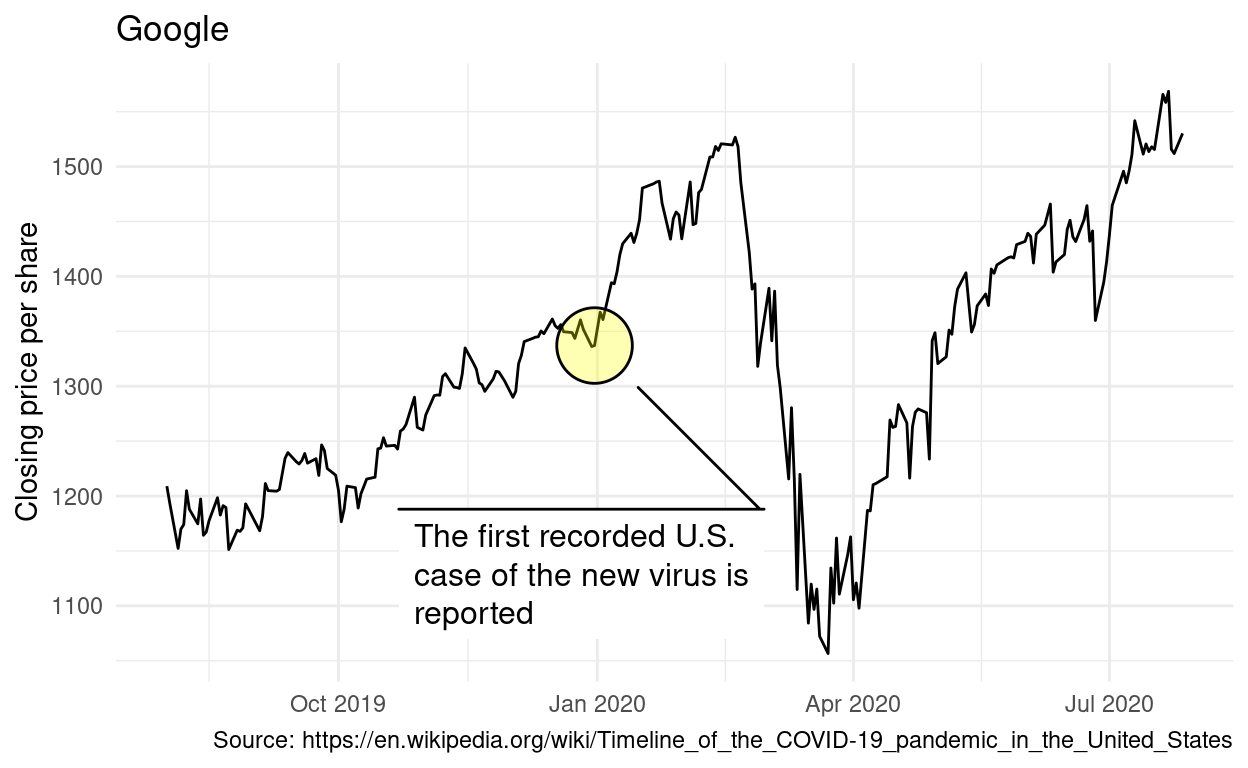# Data Visualization

Code for Quiz 9

1. Load the R package we will use.
``````library(tidyverse)
library(echarts4r)
library(ggforce)
library(tidyquant)
theme_set(theme_minimal())
``````
1. Quiz questions
• Replace all the instances of ‘SEE QUIZ’. These are inputs from your moodle quiz.

• Replace all the instances of ‘???’. These are answers on your moodle quiz.

• Run all the individual code chunks to make sure the answers in this file correspond with your quiz answers

• After you check all your code chunks run then you can knit it. It won’t knit until the ??? are replaced

• The quiz assumes that you have watched the videos, downloaded (to your examples folder) and worked through the exercises in exercises_slides-73-108.Rmd. Knitted file is here.

# Question: e_charts-1

• Create a bar chart that shows the average hours Americans spend on five activities by year. Use the timeline argument to create an animation that will animate through the years.

• spend_time contains 10 years of data on how many hours Americans spend each day on 5 activities

• read it into `spend_time`

``````spend_time  <- read_csv("https://estanny.com/static/week8/spend_time.csv")
``````

e_charts-1

Start with `spend_time`

• THEN group_by `year`

• THEN create an e_chart that assigns `activity` to the x-axis and will show activity by `year` (the variable that you grouped the data on)

• THEN use `e_timeline_opts` to set autoPlay to TRUE

• THEN use `e_bar` to represent the variable avg_hours with a bar chart

• THEN use `e_title` to set the main title to ‘Average hours Americans spend per day on each activity’

• THEN remove the legend with `e_legend`

``````spend_time  %>%
group_by(year)  %>%
e_charts(x =activity , timeline = TRUE) %>%
e_timeline_opts(autoPlay = TRUE)  %>%
e_bar(serie = avg_hours)  %>%
e_title(text ='Average hours Americans spend per day on each activity')  %>%
e_legend(show = FALSE )
``````

# Question: e_charts-2

Create a line chart for the activities that American spend time on.

Start with `spend_time`

• THEN use `mutate` to convert `year` from an number to a string (year-month-day) using `mutate`

• first convert `year` to a string “201X-12-31” using the function `paste` -`paste` will paste each year to 12 and 31 (separated by -)
• THEN use `mutate` to convert year from a character object to a date object using the `ymd` function from the `lubridate` package (part of the tidyverse, but not automatically loaded). `ymd` converts dates stored as characters to date objects.

• THEN `group_by` the variable `activity` (to get a line for each activity)

• THEN initiate an `e_charts` object with `year` on the x-axis

• THEN use `e_line` to add a line to the variable `avg_hours`

• THEN add a tooltip with `e_tooltip`

• THEN use `e_title` to set the main title to ‘Average hours Americans spend per day on each activity’

• THEN use `e_legend(top = 40)` to move the legend down (from the top)

``````spend_time  %>%
mutate(year = paste(year, "12","31", sep = "-"))  %>%
mutate (year = lubridate::ymd(year))  %>%
group_by(activity)  %>%
e_charts(x  = year)  %>%
e_line(serie = avg_hours)  %>%
e_tooltip()  %>%
e_title(text = 'Average hours Americans spend per day on each activity')  %>%
e_legend(top = 40)
``````

# Question - modify slide 82

• Create a plot with the `spend_time` data

• assign `year` to the x-axis

• assign `avg_hours` to the y-axis

• assign `activity` to color

• ADD points with `geom_point`

• ADD `geom_mark_ellipse`

• filter on activity == “leisure/sports”

• description is “Americans spend the most time on leisure/sport”

``````ggplot(spend_time, aes(x = year, y = avg_hours, color = activity,)) +
geom_point() +
geom_mark_ellipse(aes(filter = activity == "leisure/sports",
description= "Americans spend on average more time each day on leisure/sports than the other activities"))
``````# Question:tidyquant

Modify the tidyquant example in the video

Retrieve stock price for Amazon, ticker: GOOG, using `tq_get`

• from 2019-08-01 to 2020-07-28

• assign output to `df`

``````df  <-tq_get("GOOG", get = "stock.prices",
from = "2019-08-01", to = "2020-07-28" )
``````

Create a plot with the `df` data

• assign `date` to the x-axis

• assign `close` to the y-axis

• ADD a line with with `geom_line`

• ADD `geom_mark_ellipse`

• filter on a date to mark. Pick a date after looking at the line plot. Include the date in your Rmd code chunk.

• include a description of something that happened on that date from the pandemic timeline. Include the description in your Rmd code chunk

• fill the ellipse yellow

• ADD `geom_mark_ellipse`

• filter on the date that had the minimum close price. Include the date in your Rmd code chunk.

• include a description of something that happened on that date from the pandemic timeline. Include the description in your Rmd code chunk

• color the ellipse red

• ADD `labs`

``````ggplot(df, aes(x = date, y = close)) +
geom_line() +
geom_mark_ellipse(aes(
filter  = date == "2019-12-31",
description = "The first recorded U.S. case of the new virus is reported"
), fill  = "yellow",) +
geom_mark_ellipse(aes(
filter  = date == "2020-07-30",
description = "Justice Dept creates task force"
), color = "red", ) +
labs(